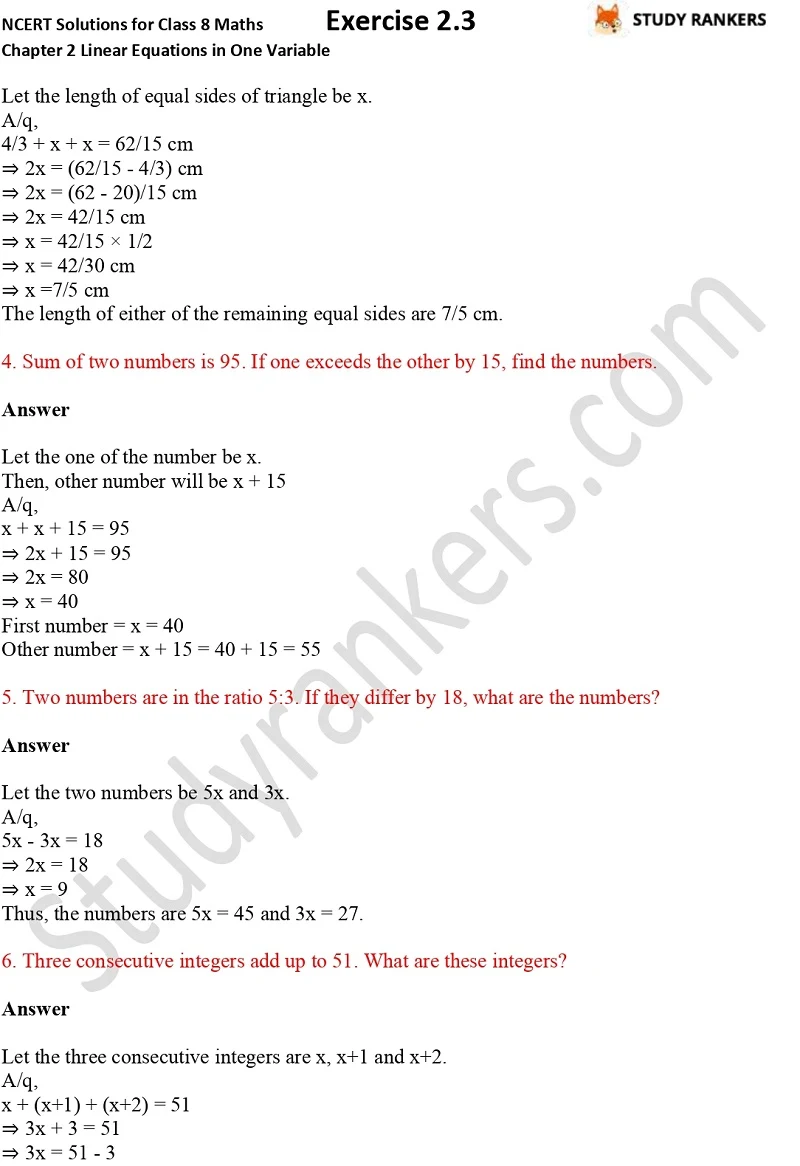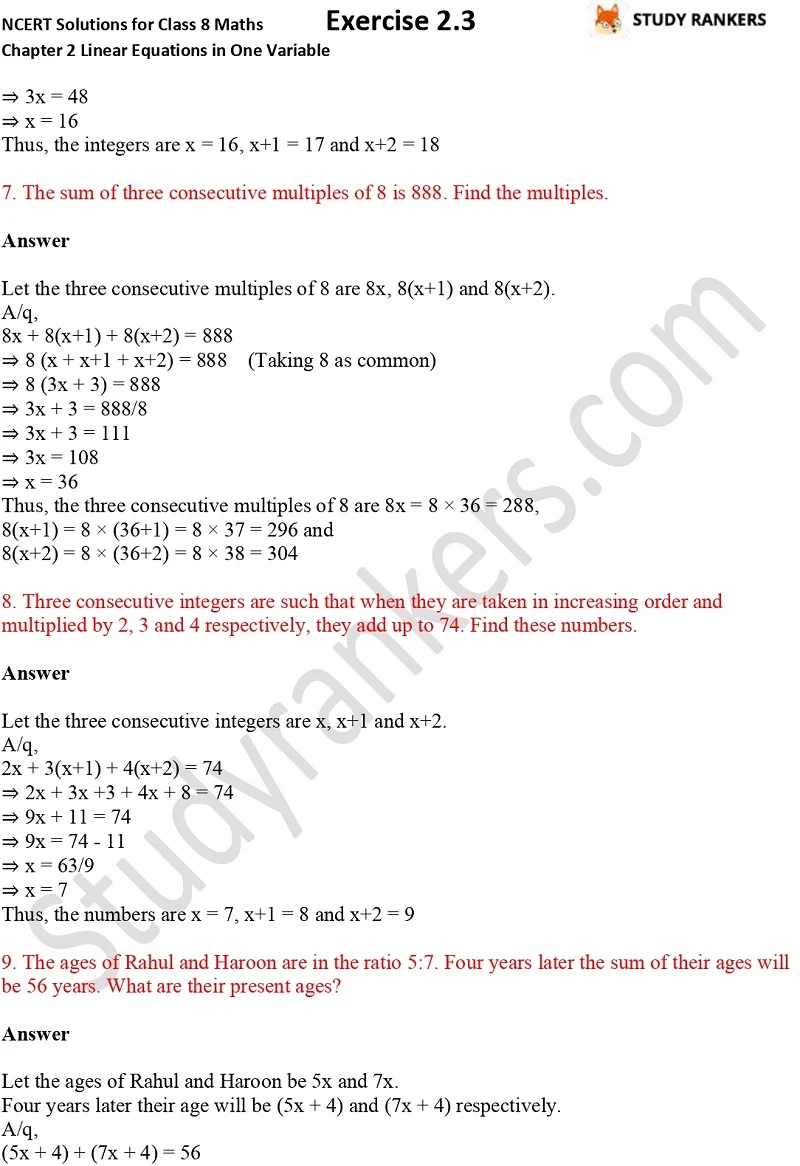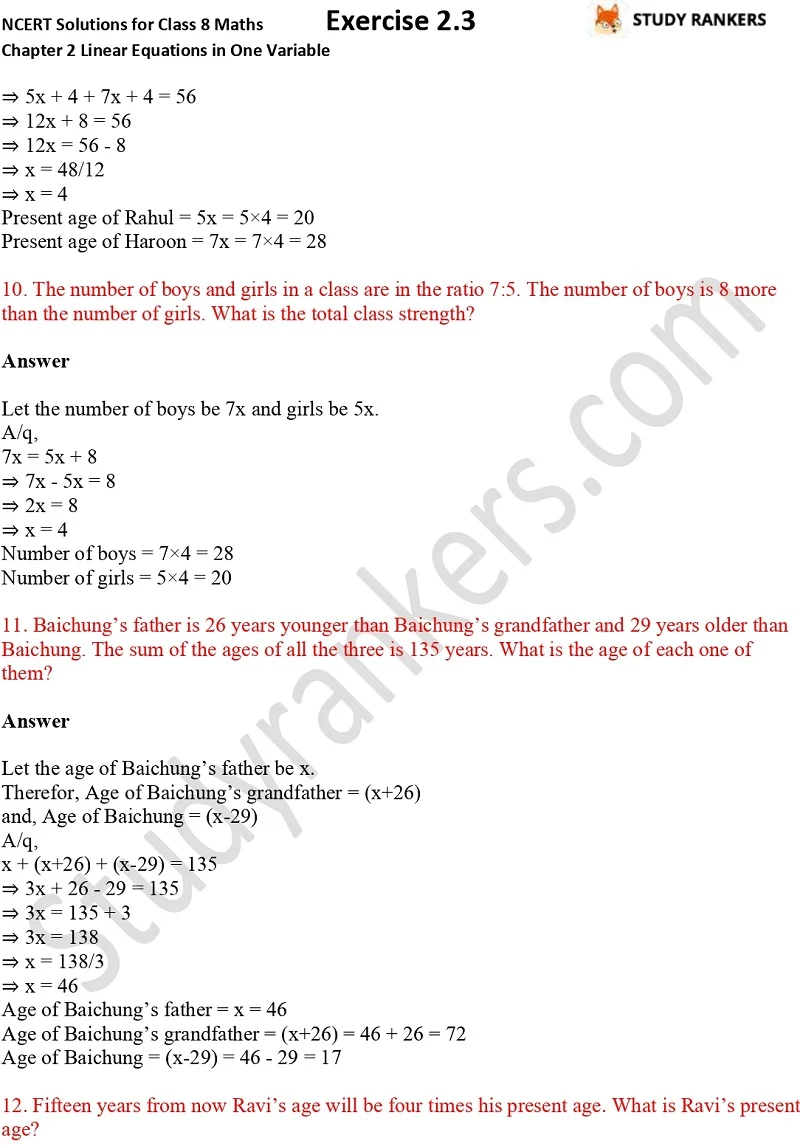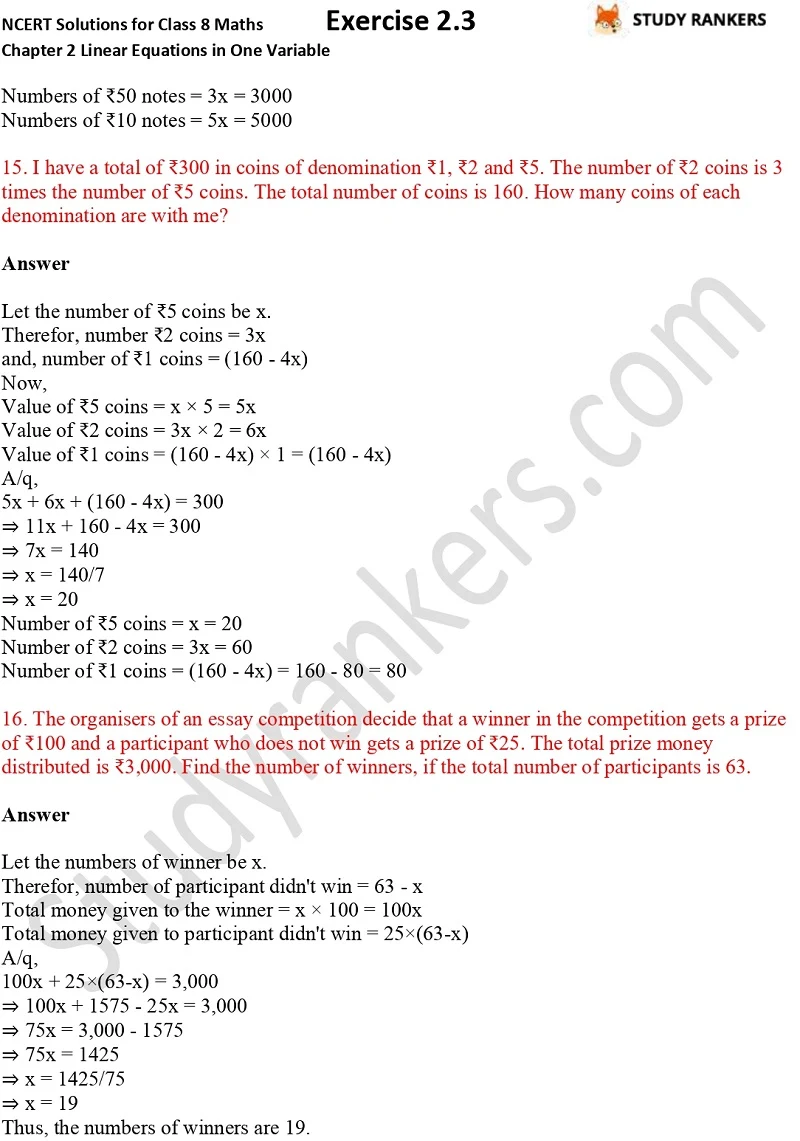## NCERT Solutions for Class 8 Maths Chapter 2 Linear Equations in One Variable Exercise 2.3

You can find for Chapter 2 Linear Equations in One Variable Exercise 2.3 NCERT Solutions Class 8 Maths here which will help you in completing your homework on time and preparing for examinations. These NCERT Solutions for Class 8 Maths will guide in knowing the important concepts and points of the chapter. It will be useful in knowing how to apply formulas and solving the questions easily. These NCERT questions and answers are updated as per the latest syllabus.

There are only one question in the exercise 2.3 in which you have to solve the given equations.